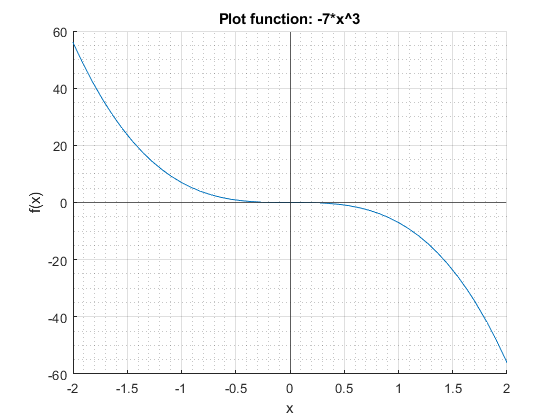1 Matlab Graph One Variable Function

Go to the MLX, M, PDF, or HTML version of this file. Go back to fan’s MEconTools Package, Matlab Code Examples Repository (bookdown site), or Math for Econ with Matlab Repository (bookdown site).

1.1 fplot a Function of X

Given a cubic (polynomial) function, graph it using the fplot function, between some values along the domain of the function. This function is defined everywhere along the real-line. Note that fplot automatically resizes the y-scale to show the full plot clearly.

% close all
figure();
hold on;

% Define a function
syms x
f_x = -7*x^(3);

% Set bounds on the domain
fl_x_lower = -2;
fl_x_higher = 2;

% Graph
fplot(f_x, [fl_x_lower, fl_x_higher])

xline(0);
yline(0);

% Title and y and y-able
title(['Plot function: ' char(f_x)],'Interpreter',"none");
ylabel('f(x)');
xlabel('x');

grid on;
grid minor;Plot a rational function, that is a function of polynomials.

% close all
figure();
hold on;

% Define a function
syms x
f_x = (x)/(x^2 + 1);

% Set bounds on the domain
fl_x_lower = -2;
fl_x_higher = 2;

% Graph
fplot(f_x, [fl_x_lower, fl_x_higher])